# Redis 源码解析 4：字典 dict

Redis 中，字典是基础结构。Redis 数据库数据、过期时间、哈希类型都是把字典作为底层结构。

# 字典的结构

## 哈希表

typedef struct dictht {
// 哈希表数组，俗称的哈希桶(bucket)
dictEntry **table;
// 哈希表的长度
unsigned long size;
// 哈希表的长度掩码，用来计算索引值，保证不越界。总是 size - 1
// h = dictHashKey(ht, he->key) & n.sizemask;
// 哈希表已经使用的节点数
unsigned long used;
} dictht;

• table 是一个哈希表数组，每个节点的实现在 dict.h/dictEntry，每个 dictEntry 保存一个键值对。
• size 属性记录了向系统申请的哈希表的长度，不一定都用完，有预留空间的。
• sizemask 属性主要是用来计算 索引值 = 哈希值 & sizemask，这个索引值决定了键值对放在 table 的哪个位置。它的值总是 size - 1，其实我有点不明白为啥计算的时候不直接用 size - 1，知道的大佬请明示。
• used 属性用来记录已经使用的节点数，size - use 就是未使用的节点啦。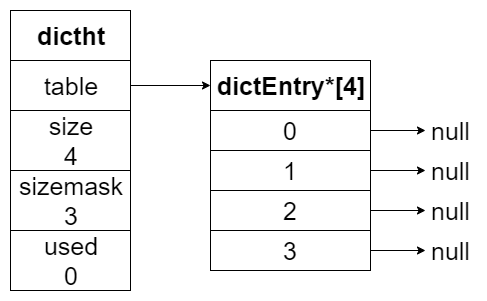## 哈希节点

typedef struct dictEntry {
// 键
void *key;
// 值
union {
void *val;
uint64_t u64;
int64_t s64;
double d;
} v;
// 下一个哈希节点，用于哈希冲突时拉链表用的
struct dictEntry *next;
} dictEntry;


next 指针是用于当哈希冲突的时候，可以形成链表用的。后续会将

## 字典

Redis 的字典实现在： dict.h/dict

typedef struct dict {
// 哈希算法
dictType *type;
// 私有数据，用于不同类型的哈希算法的参数
void *privdata;
// 两个哈希表，用两个的原因是 rehash 扩容缩容用的
dictht ht;
// rehash 进行到的索引值，当没有在 rehash 的时候，为 -1
long rehashidx; /* rehashing not in progress if rehashidx == -1 */
// 正在跑的迭代器
unsigned long iterators; /* number of iterators currently running */
} dict;

// dictType 实际上就是哈希算法，不知道为啥名字叫 dictType
typedef struct dictType {
// hash方法，根据 key 计算哈希值
uint64_t (*hashFunction)(const void *key);
// 复制 key
void *(*keyDup)(void *privdata, const void *key);
// 复制 value
void *(*valDup)(void *privdata, const void *obj);
// key 比较
int (*keyCompare)(void *privdata, const void *key1, const void *key2);
// 销毁 key
void (*keyDestructor)(void *privdata, void *key);
// 销毁 value
void (*valDestructor)(void *privdata, void *obj);
} dictType;


dictType 属性表示字典类型，实际上这个字典类型就是一组操作键值对算法，里面规定了很多函数。
privdata 则是为不同类型的 dictType 提供的可选参数。

dict.c

// 创建字典，这里有 type 和 privdata 可以传
dict *dictCreate(dictType *type, void *privDataPtr) {
dict *d = zmalloc(sizeof(*d));
_dictInit(d,type,privDataPtr);
return d;
}

// 初始化字典
int _dictInit(dict *d, dictType *type, void *privDataPtr) {
_dictReset(&d->ht);
_dictReset(&d->ht);
d->type = type;
d->privdata = privDataPtr;
d->rehashidx = -1;
d->iterators = 0;
return DICT_OK;
}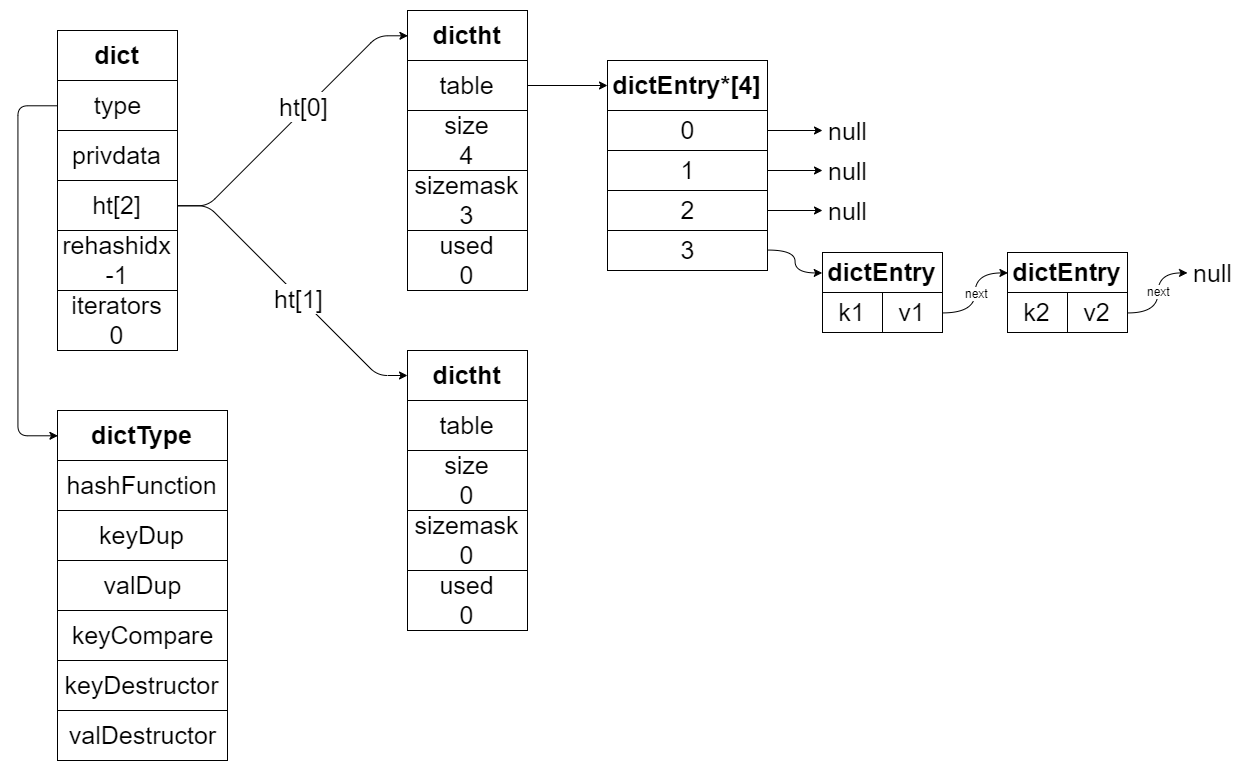# 哈希算法

// 使用哈希字典里面的哈希算法，算出哈希值
hash = dict->type->hashFunction(key)
// 通过索引值，定位哈希节点
he = d->ht[table].table[idx];


## 哈希冲突

Redis 解决哈希冲突的方法是：拉链法。就是每个哈希节点后面有个 next 指针，当发现计算出的索引值对应的位置有其他节点，那么直接加在前面节点后即可，这样就形成了一个链表。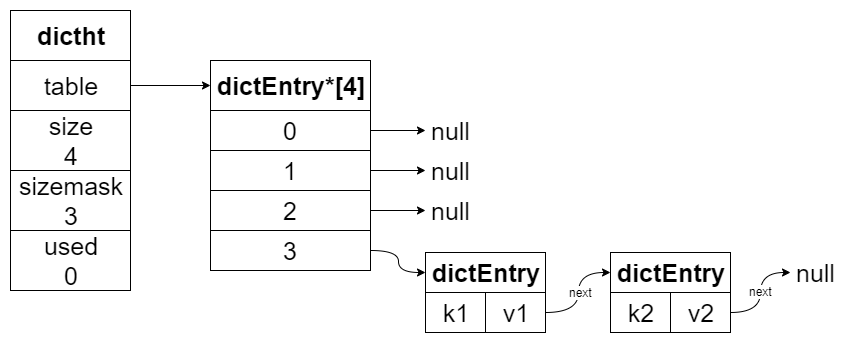## rehash

Redis 通过 ht 和 ht 来完成 rehash 的操作，步骤如下：

1. 为 ht 分配空间，分配的空间长度有两种情况：
• 扩容：第一个大于等于 ht.used * 2$2^n$ 的数，例如 ht.used = 3，那么分配的是距离 6 最近的 $2^3=8$
• 缩容：第一个大于等于 ht.used / 2$2^n$ 的数，例如 ht.used = 6，那么分配的是距离 3 最近的 $2^2=4$
2. 将 h 上的键值对都迁移到 h，迁移的时候都是重新计算索引值的。由于 h 的长度较长，之前在 h 拉链的元素大概率会被分到不同的位置。
3. ht 所有的键值对迁移完之后，h 释放，然后 h = h，并把 h 清空，为下次 rehash 准备

## 渐进式 rehash

1. 为 h 开辟空间，字典同时持有 h 和 h
2. 字典中的 rehashidx 维护了 rehash 的进度，设置为 0 的时候，开始 rehash
3. 字典每次增删改查的时候，除了完成指定操作之外，还会顺带把 rehashidx 上的整条链表迁移到 h 中。迁移完之后 rehashidx + 1
4. 随着字典的不断读取、操作，最终 h 上的所有键值对都会迁移到 h 中。全部迁移完成之后 rehashidx = -1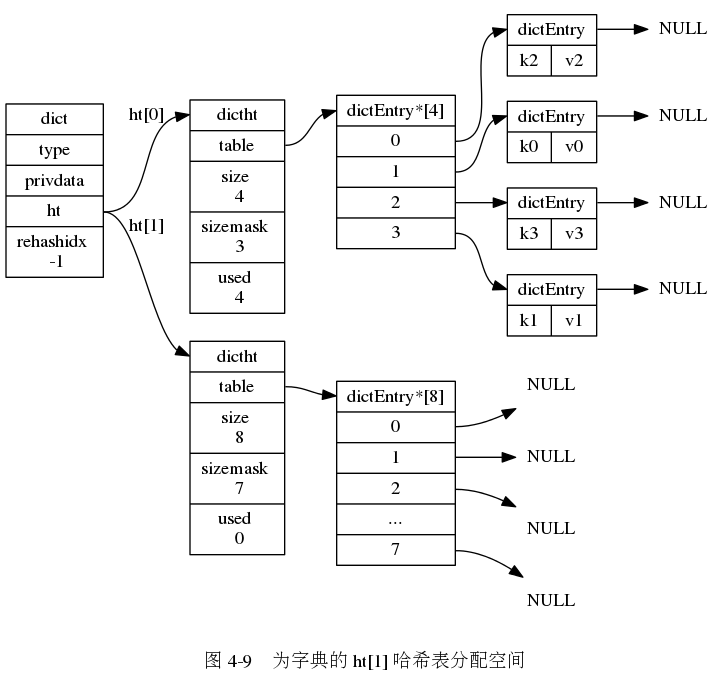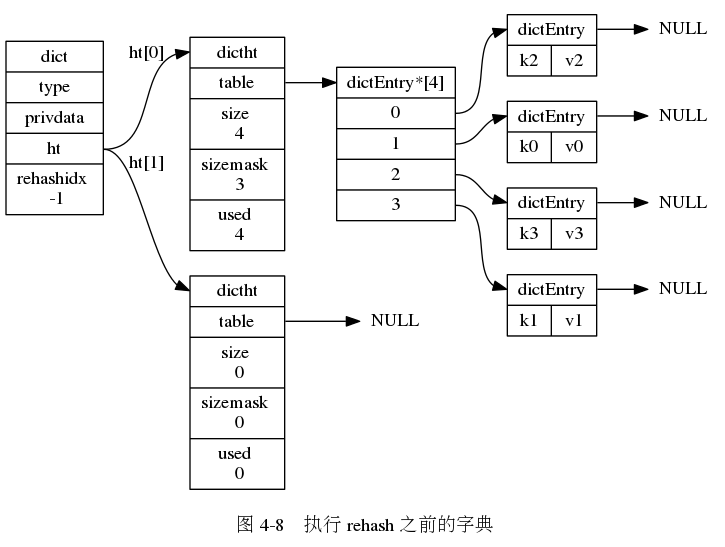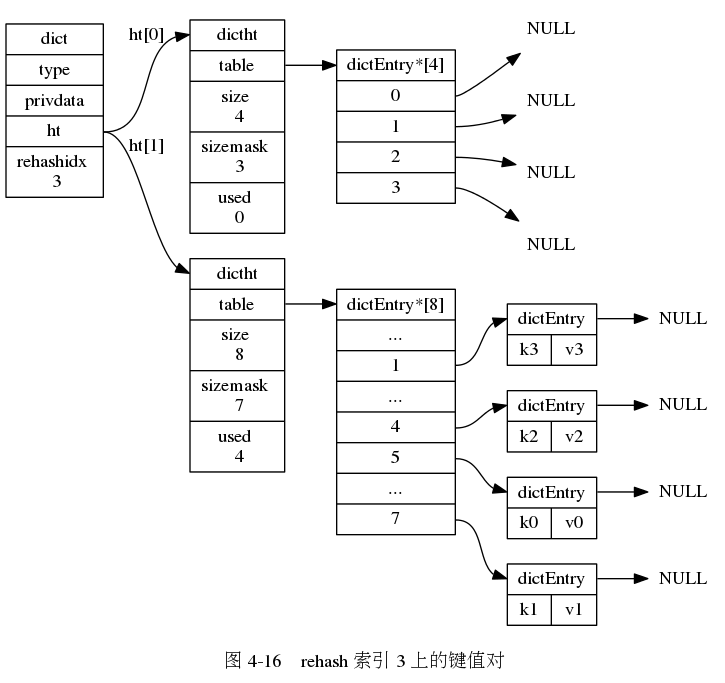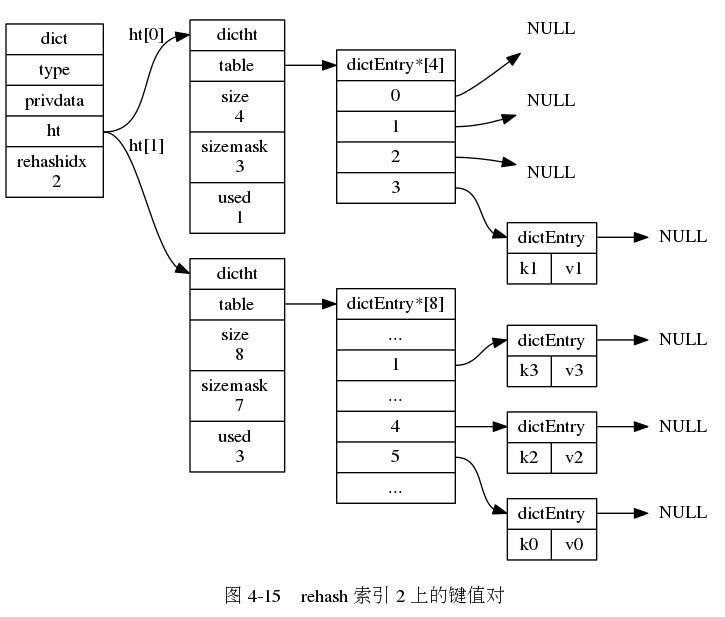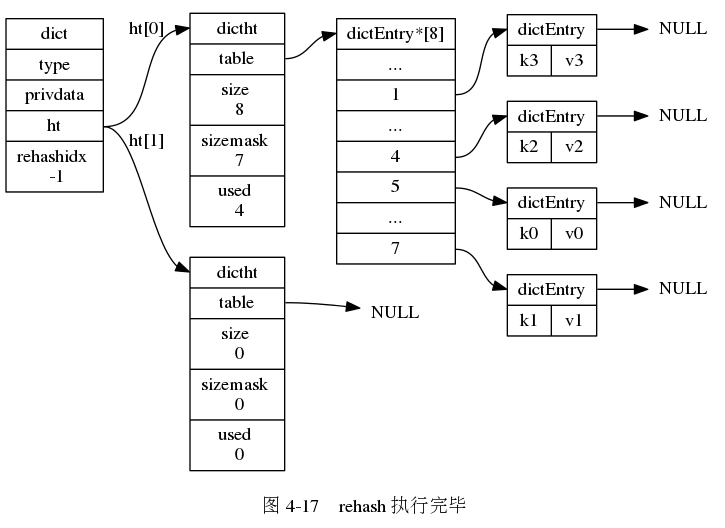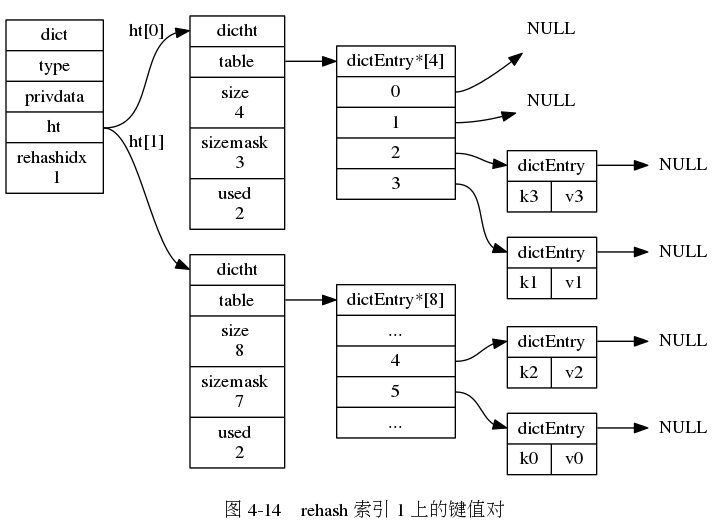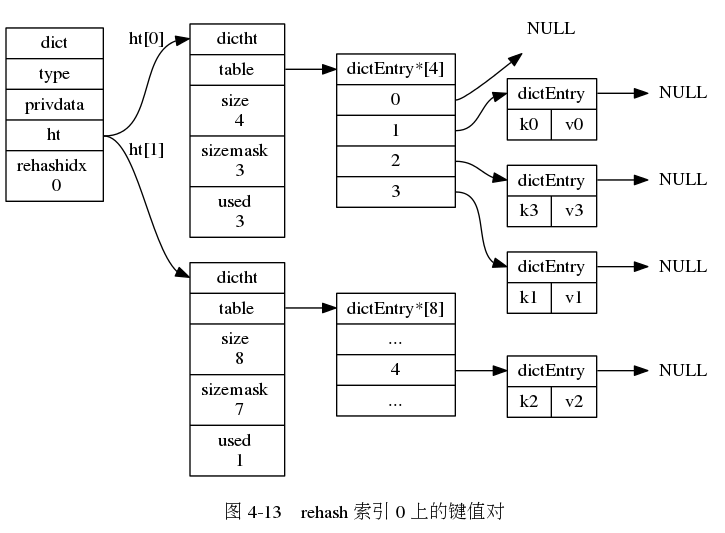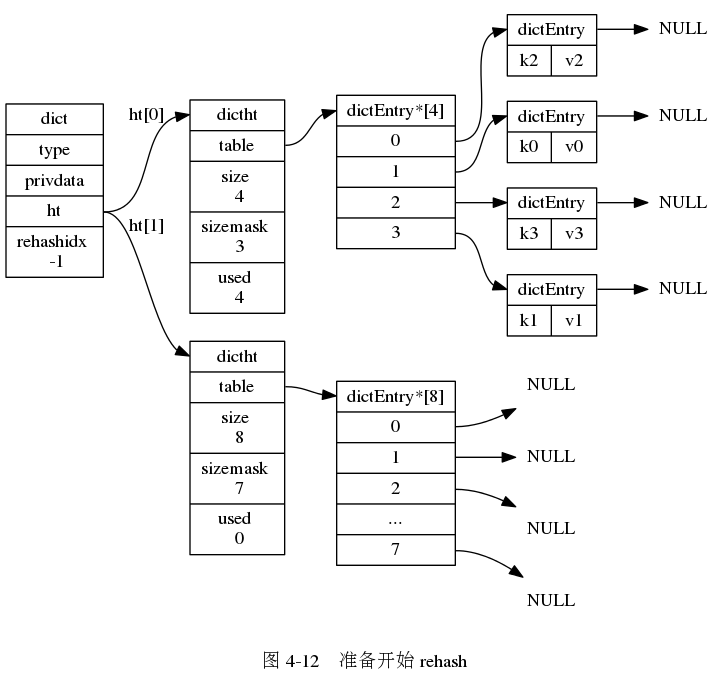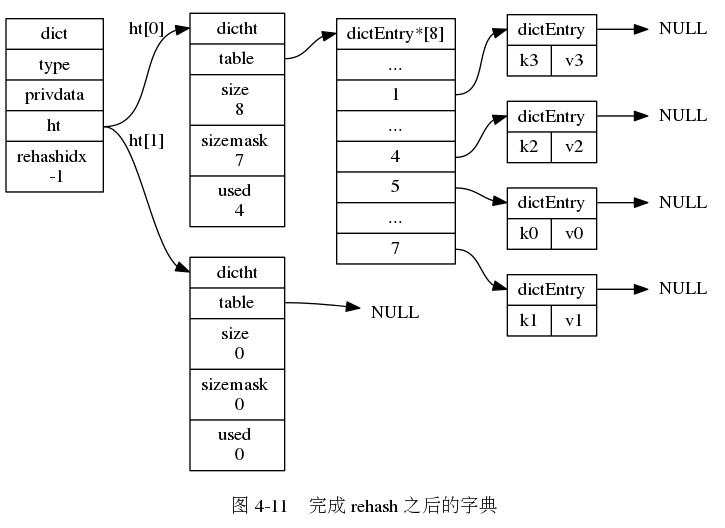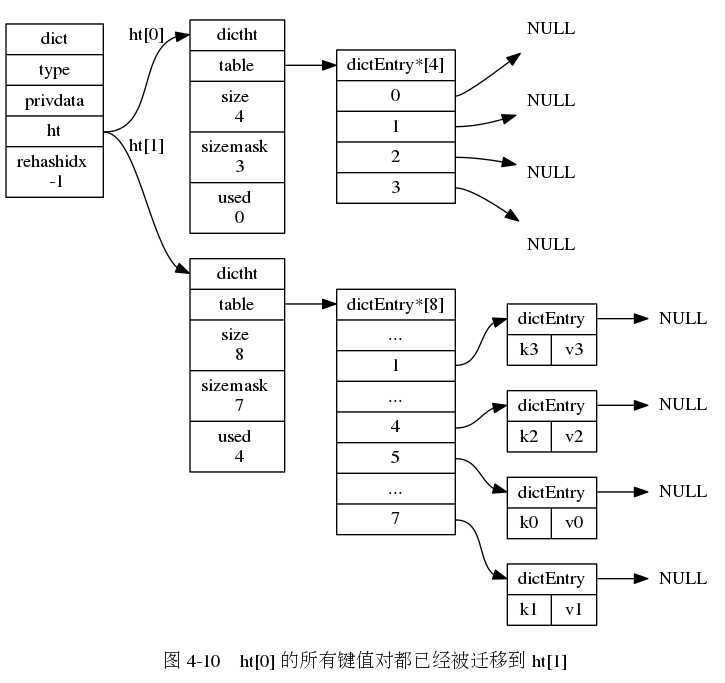# 字典的常用操作

## 1. 查找节点

#define dictHashKey(d, key) (d)->type->hashFunction(key)
// ...
dictEntry *dictFind(dict *d, const void *key) {
dictEntry *he;
uint64_t h, idx, table;
// 空字典直接返回 NULL
if (dictSize(d) == 0) return NULL;
// 如果状态是正在 rehash，则渐进式 rehash 一步
if (dictIsRehashing(d)) _dictRehashStep(d);
// 调用字典的哈希算法进行哈希，算出哈希码
h = dictHashKey(d, key);

for (table = 0; table <= 1; table++) {
// 算出在哈希桶中的 index
// 找到对应的哈希槽
he = d->ht[table].table[idx];
// 哈希槽是个链表
while(he) {
// 遍历链表，找到跟 key 相等的节点
if (key==he->key || dictCompareKeys(d, key, he->key))
return he;
he = he->next;
}
// 如果没有在 rehash，就不找 ht 了
if (!dictIsRehashing(d)) return NULL;
}
return NULL;
}


## 2. 添加节点

int dictAdd(dict *d, void *key, void *val) {
// 底层添加节点的方法
dictEntry *entry = dictAddRaw(d, key, NULL);
if (!entry) return DICT_ERR;
// 给节点设置值
dictSetVal(d, entry, val);
return DICT_OK;
}

// 添加节点的方法
dictEntry *dictAddRaw(dict *d, void *key, dictEntry **existing) {
long index;
dictEntry *entry;
dictht *ht;

// 国际惯例，rehash 一波
if (dictIsRehashing(d)) _dictRehashStep(d);

// 查看插入的 key 是否已经存在，已经在则返回 NULL
if ((index = _dictKeyIndex(d, key, dictHashKey(d,key), existing)) == -1)
return NULL;

// 如果正在 rehash，那就放在 ht 就行了
ht = dictIsRehashing(d) ? &d->ht : &d->ht;
entry = zmalloc(sizeof(*entry));
// 把节点放到对应的哈希槽
entry->next = ht->table[index];
ht->table[index] = entry;
ht->used++;

// 节点的 key 赋值
dictSetKey(d, entry, key);
return entry;
}


## 3. 删除节点

static dictEntry *dictGenericDelete(dict *d, const void *key, int nofree) {
uint64_t h, idx;
dictEntry *he, *prevHe;
int table;

// 如果是空的，返回
if (d->ht.used == 0 && d->ht.used == 0) return NULL;

// 国际惯例，rehash 一波
if (dictIsRehashing(d)) _dictRehashStep(d);
// 根据字典的哈希算法，算出哈希码
h = dictHashKey(d, key);

for (table = 0; table <= 1; table++) {
// 算出索引
// 找到哈希槽
he = d->ht[table].table[idx];
prevHe = NULL;
while(he) {
if (key==he->key || dictCompareKeys(d, key, he->key)) {
/* Unlink the element from the list */
if (prevHe)
prevHe->next = he->next;
else
d->ht[table].table[idx] = he->next;
if (!nofree) {
// 释放节点。
dictFreeKey(d, he);
dictFreeVal(d, he);
zfree(he);
}
d->ht[table].used--;
return he;
}
prevHe = he;
he = he->next;
}
if (!dictIsRehashing(d)) break;
}
}


nofree 参数的意思是"是否要释放找到的节点的内存"，为什么需要这个参数呢？

int zsetDel(robj *zobj, sds ele) {
if (zobj->encoding == OBJ_ENCODING_ZIPLIST) {
// ...
} else if (zobj->encoding == OBJ_ENCODING_SKIPLIST) {
// skiplist 编码
zset *zs = zobj->ptr;
dictEntry *de;
double score;

// 找到要删除的哈希节点，但是先不释放内存
if (de != NULL) {
// 拿到节点的分数，用于删掉链表节点
/* Get the score in order to delete from the skiplist later. */
score = *(double*)dictGetVal(de);
// 释放节点内存